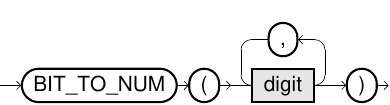# BIT_TO_NUM

## Purpose

This function creates a numerical value out of a list of single bits.

## Syntax

bit_to_num::=## Usage Notes

• Each function argument must evaluate to 0 or 1.
• The first argument is interpreted as the biggest bit.
• The number of arguments is limited to 64, which means you can create positive numbers between 0 and 18446744073709551615.

## Examples

``SELECT BIT_TO_NUM(1,1,0,0);``